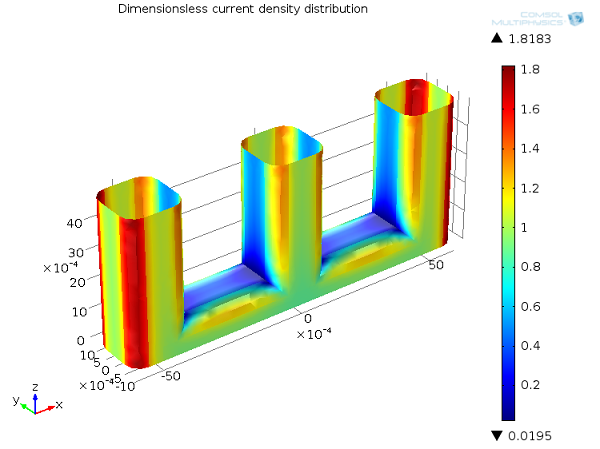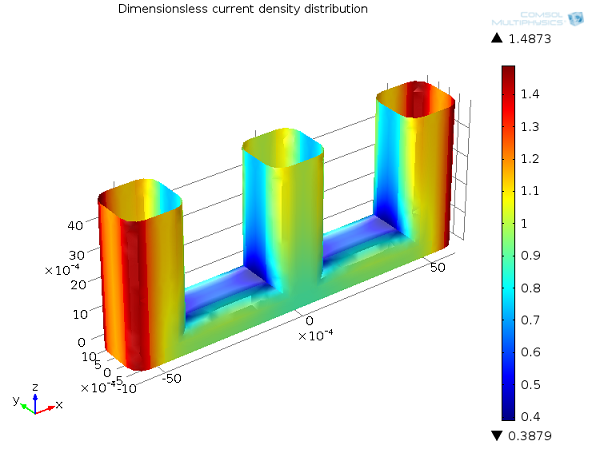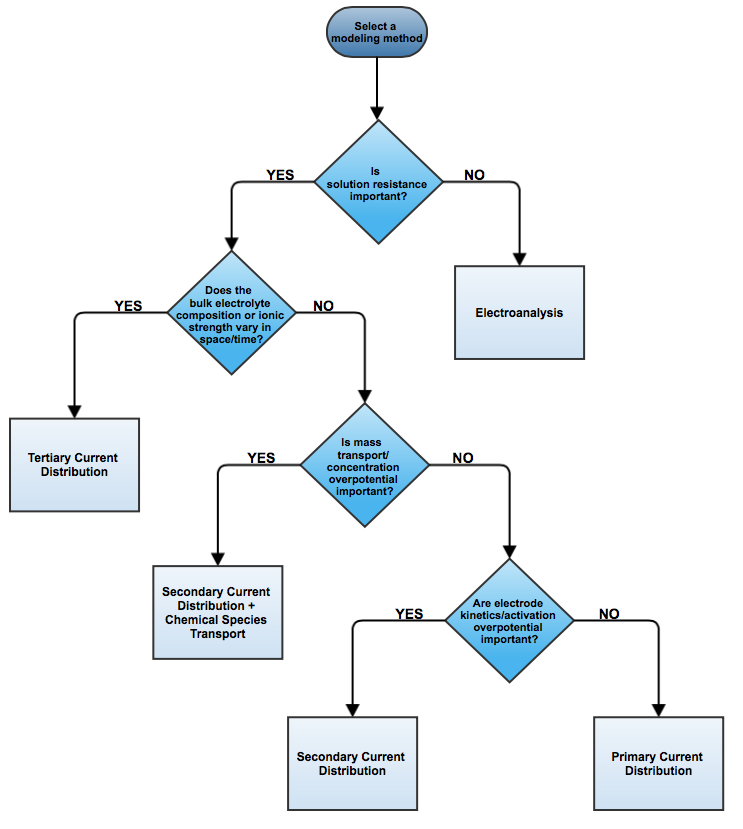# 如何选择正确的电流分布接口？

2014年 2月 10日

### 三种电流分布接口### 回顾基本方程

Nernst-Planck 方程：

(1)

\textbf{N}_i = -D_i\nabla c_i-z_i u_{m,i} F c_i\nabla \phi_l+c_i\textbf{u}

(2)

\textbf{i}_l = -F \left(\nabla \sum_i z_iD_i c_i\right)-F^2\nabla \phi_l \sum_i z^2_i u_{m,i} c_i+\textbf{u}F\sum_i z_ic_i

\nabla\cdot\mathbf{i}_l=Q_l

### 一次电流分布

COMSOL Multiphysics 的一次电流分布 接口定义了两个相关变量：一个是电解质电势（\phi_l\），另一个是电极电势（\phi_s\）。基于上述的一次电流分布假设，可得以下方程:### 二次电流分布

(3)

i_{loc,m} = i_{0,m}\left(e^\frac{\alpha_{a,m} F \eta_m}{RT}-e^\frac{-\alpha_{c,m} F \eta_m }{RT}\right)

-\textbf{i}_s \cdot \textbf{n} = \textbf{i}_l \cdot \textbf{n} = i_\mathrm{DL} + \sum_m{i_{loc,m}}### 三次电流分布

COMSOL 软件中的三次电流分布 接口求解了电解质电位（\phi_l\）、电极电位（\phi_s\）和一组物质浓度 c_i。根据上述假设，可得到以下方程：### 还有哪些其他选项？

#### 电分析